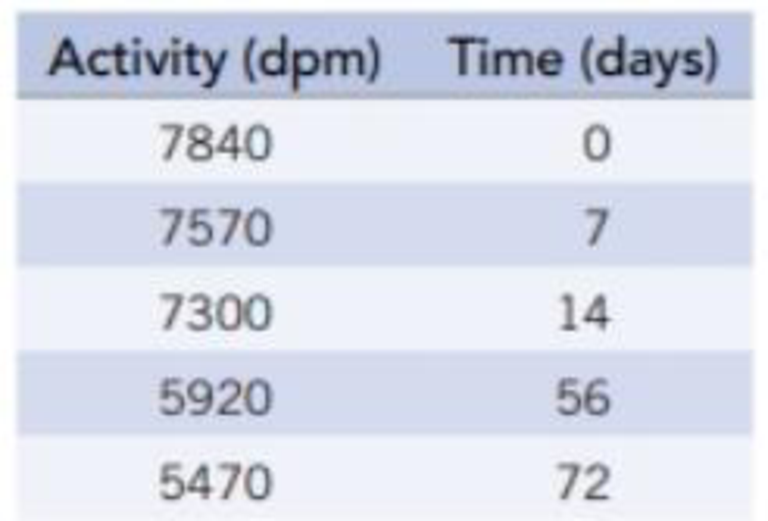Chapter 25, Problem 67IL

Chapter
Section
Textbook Problem

The isotope of polonium that was most likely isolated by Marie Curie in her pioneering studies is polonium-210. A sample of this element was prepared in a nuclear reaction. Initially, its activity (α emission) was 7840 dpm. Measuring radioactivity over time produced the data below. Determine the half-life of polonium-210.Interpretation Introduction

Interpretation:

The half-life of polonium-210 has to be determined.

Concept Introduction:

Radiocarbon dating: The dynamic equilibrium exists in all living organism by exhaling or inhaling, maintain the same ratio of 12C and 14C that takes in. when the living organism dies, the intake of 14C stops and it’s the ratio no longer exhibits equilibrium; undergoes radioactive decay.

Half-life period: The time required to reduce to half of its initial value.

Formula used to calculate half-life:

t1/2=0.693kwhere,kis rate constant.(or)ln[N]t- ln[N]0= -kt

Explanation

Activities for polonium-210 are 7840, 7570,7300,5920,5470.

Time for polonium-210 is 0, 7, 14, 56 and72.

The seventh activity;

ln[AAo]= -k(t)ln= -k(t)ln=0.965= -k(7)-0.0356k=0.005

The fourteenth activity;

ln[AAo] = -k(t)ln = -k(t)ln=0.9311 = -k(14) -0.07138k = 0.005

The fifty sixth activity

ln[AAo]= -k(t)ln= -k(t)ln = 0

Still sussing out bartleby?

Check out a sample textbook solution.

See a sample solution

The Solution to Your Study Problems

Bartleby provides explanations to thousands of textbook problems written by our experts, many with advanced degrees!

Get Started

Cod is one of the richest sources of the beneficial fatty acids, EPA and DHA. T F

Nutrition: Concepts and Controversies - Standalone book (MindTap Course List)

Show that 1 cubic meter contains 1000 L.

An Introduction to Physical Science

Describe what is meant by the term primary structure of proteins.

Chemistry for Today: General, Organic, and Biochemistry